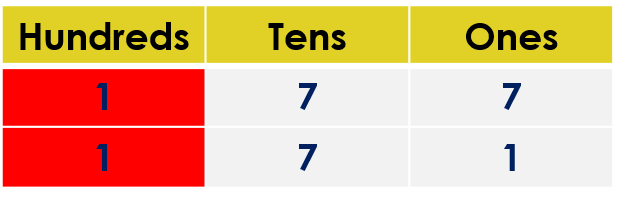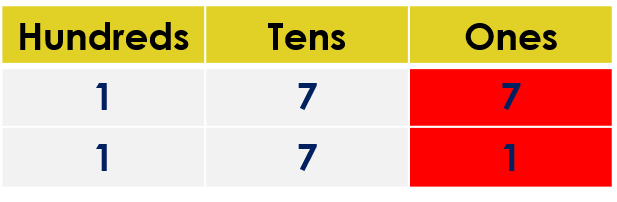1
visibility
Fill in the blank space with the correct sign. Use either <, > or =.
177$\framebox[0.5in]{}$  171

It is easy!

• Compare the hundreds digits.
• If the hundreds digits are the same, then compare the tens digits.
• If tens digits are the same, then compare the ones digits.

Solution:

Observe that both numbers have three digits.

177 ____ 171

Comparing the hundreds digits:We observe that both digits are the same.

Comparing the tens digits:We observe that both digits are the same.

Comparing the ones digits:We observe that both digits are NOT the same.

• The ones digit in the number 177 is 7.
• The ones digit in the number 171 is 1.

But, 7 is greater than 1.

So, 177 is greater than 171.

That is, 177 > 151.